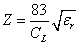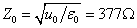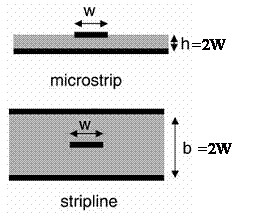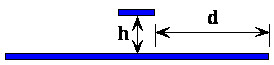Register & Pay for Your 1st Order : Enjoy 10% OFF Discount for Next !

# The role and location of characteristic impedance in signal integrity

1 1 63

When the signal propagates on the transmission line, the transient impedance sensed by the signal is related to the capacitance per unit length and the dielectric constant of the material.

Can be expressed as:If the thickness and width of the lines on the PCB are constant and the distance between the trace and the return plane is constant, the transient impedance experienced by the signal is unchanged and the transmission line is uniform. For a uniform transmission line, a constant transient impedance describes the characteristics of the transmission line, called the characteristic impedance.

If the thickness of the line on the PCB increases or the width increases, the capacitance per unit length increases, and the characteristic impedance becomes smaller. Similarly, the distance between the trace and the return plane is reduced, the capacitance is increased, and the characteristic impedance is also reduced.

A very important characteristic impedance is the characteristic impedance of free space, also known as the wave impedance of free space, which is very important in EMC. The free space characteristic impedance is.

For a typical FR4 board PCB, the typical structure of the 50Ω characteristic impedance is shown. For the microstrip line, the line width W is twice the thickness h of the medium. For a strip line, the total thickness b of the media on both sides of the line is twice the line width W.On the PCB board of FR4 board, another characteristic of the characteristic impedance transmission line is:

Unit length capacitance = 3.3pF / in

Unit length capacitance = 8.3nH / in

Characteristic impedance, the role and position of characteristic impedance in signal integrityUnderstanding these special characteristic impedances has certain reference significance for designing circuit boards, which allows us to have an intuitive understanding before making circuits.

Comments(1)
Please log in or sign to comment